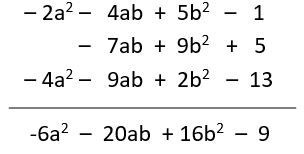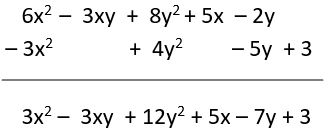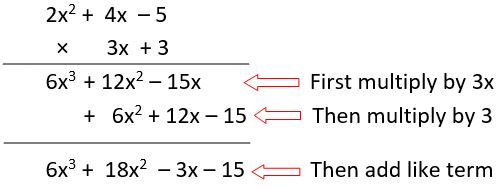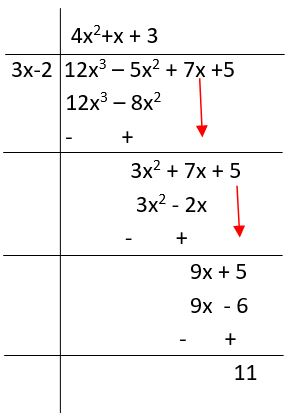## LetsPlayMaths.Com

WELCOME TO THE WORLD OF MATHEMATICS

# Class-8 Algebraic Expressions

Introduction to Algebra

Constant and Variables

Terms of Algebraic Expression

Coefficient of a Term

Like and Unlike Terms

Polynomials in One Variable

Polynomial in Two or More Variables

Linear polynomial

Degree of a Polynomial

Arithmetic Operation of Algebraic Expression

Addition of Algebraic Expression

Subtraction of Algebraic Expression

Multiplication of Algebraic Expression

Division of Algebraic Expressions

Algebraic Identity

Standard Identities

Algebraic Expressions Test

Algebraic Expressions Worksheets

## Introduction to Algebra

It is branch of mathematics in which we use literal numbers and statements symbolically. Literal numbers can be positive or negative, they are variables.

## Constant and Variables

In algebra, we use two types of symbols, that is constants and variables.

A symbol which has a fixed value is known as Constant. For example, 6, -2, 0, 35, 53, etc. are constant.

A symbol (letter) which can be given various numerical values is known as variable. As variables are usually denoted by letters, so it is also known as literal / literal numbers. Let's have a look at some examples.

In 3n, 3 is constant and 'n' is variable. But together 3n is a variable.
When n = 1, 3n = 3 x 1 = 3
When n = 2, 3n = 3 x 2 = 6
When n = 3, 3n = 3 x 3 = 9
So, when 'n' takes various values , 3n also change accordingly.
Similarly, n + 2, n −3, 5 −n , 2n and n5 are variables.

Hence a combination of constant and variable is also a variable.

### Algebraic Expressions

A collection of constants and literals (variables) connected by one or more arithmetic operations is called an algebraic expression. Let's see some examples.

Expression Formation
a + 3 3 is added 'a'
b − 6 6 is subtracted from b
2n 2is multiplied by 'n'
n7 'n' divided by 7
−5y −5 is multiplied by 'y'
2n + 3 First 'n' is multiplied by 2 then 3 is added to the result

## Terms of Algebraic Expression

The various part of an algebraic expression separated by +/− sign are known as terms of the algebraic expression. Various types of algebraic expression are:

1. Monomial − An algebraic expression having one term
2. Binomial − An algebraic expression having two terms
3. Trinomial − An algebraic expression having three terms
4. Multinomial − An algebraic expression having two or more terms

Expression No of terms Name Terms
-7a2b3 1 Monomial -7a2b3
3a + 5b 2 Binomial 3a, 5b
3n + 7m2 + 25 3 Trinomial 3n, 7m2, 25
2x5 − 5x2 + 4 − y2 4 Multinomial 2x5,−5x2, + 4, -y2

## Coefficient of a Term

Any factor of a term of an algebraic expression is called coefficient of the remaining factor of the term. Let's see some examples.

Example: 5abc + 4ab2 − 4

In the term 5abc,

Numerical coefficient = 5

Literal coefficient = abc

Coefficient of a = 5bc

## Like and Unlike Terms

The terms having same literal coefficients are called like terms, otherwise, the terms are called unlike terms. Let's see some examples.
2xy, 3xy, 4xy, 5xy, −xy, 23xy are like terms.
2m, 4mn, 3m2n are unlike terms.

## Polynomials in One Variable

An algebraic expression having only one variable of the form a + bx + cx2 + ..., where a, b, c, ... are constants and 'x' is a variable, is known as a polynomial in the variable 'x'. Let's see some examples.

Example 1. 3 + 4x is a polynomial in 'x' of degree 1.

Example 2. 2 − 3y + 5y2 is a polynomial in 'y' of degree 2.

## Polynomial in Two or More Variables

An algebraic expression having two or more variables called polynomial in those variables. Powers are here non negative integer.

Example 1. 8a2b3 − 5a2b + 7ab − 15 is a polynomial in two variables a and b. degree of its terms are 2+3, 2+1, 1+1, 0. So, here degree of polynomial is 5.

Example 2. 5ab22ab + 5b3 − 5 is not a polynomial as in term − (2ab) that is written as (−2a)b-1,the power of b is a negative integer.

## Linear polynomial

A polynomial of degree 1 is known as linear polynomial.

Example 1. 3a + 5, 5 − 2a are linear variable with one variable.

Example 2. 5a + 2b , 2a − 12b + 11 are all linear polynomials in two variables.

Example 3. 2a + 3b − 5c, 5a − 3b + 4c − 2 are linear polynomial in three variables.

## Degree of a Polynomial

The degree of each term in a polynomial is the sum of the exponents of variables of the term and the degree of the polynomials is the greatest of the degrees of its terms. Let's see some examples.

Example 1. Find out the degree in the given polynomial
3 + 4p

Solution. In 3 + 4p, the degree of two terms 3 and 4p are 0 and 1, respectively. The degree of the polynomials is 1.

Example 2. Find out the degree in the given polynomial
5y2 + 3y − 2

Solution. In 5y2 + 3y − 2, the degree of 3 terms 2, 1 and 0, respectively. So, the degree of the polynomial is 2.

## Arithmetic Operation of Algebraic Expression

1. Addition of algebraic expression
2. Subtraction of algebraic expression
3. Multiplication of algebraic expression
4. Division of algebraic expression

We did addition and subtraction of algebraic expression in detail in class 7. Now we recall addition and subtraction operation, and study more details about multiplication and division operation.

## Addition of Algebraic Expression

1. Horizontal method
2. Column method

### Horizontal method

In this method we collect different group of like terms and then find the sum of like terms in each group. Lets see some examples.

Example 1. Add 2a2 − 4ab + 5b2 − 1, 9b2 − 7ab + 5 and −9ab − 4a2 + 2b2 − 13

Solution. (2a2 − 4ab + 5b2 − 1) + (9b2 − 7ab + 5) + (−9ab − 4a2 + 2b2 − 13)

= 2a2 − 4ab + 5b2 − 1 + 9b2 − 7ab + 5 − 9ab − 4a2 + 2b2 − 13

= 2a2 − 4a2 + 5b2+ 9b2 + 2b2 − 4ab −7ab − 9ab −1 + 5 − 13

Add all underlined liked terms in each group and get final result.

= −2a2 + 16b2 − 20ab − 9

### Column method

In this method we write each expression to be added in separate row in such way that like term should come below other and add them. Let's see one example to understand it better.

Example 1. Add (−2a2 − 4ab + 5b2 − 1), (9b2 − 7ab + 5), and (−9ab − 4a2 + 2b2 − 13)

Solution.## Subtraction of Algebraic Expression

1. Horizontal method
2. Column method

### Horizontal method

To subtract algebraic expressions, first we should change the sign of each of algebraic expression to be subtracted and then add by horizontal method. Let's see one example to understand it better.

Example 1. Subtract (3x2 − 4y2 + 5y − 3) from (6x2 − 3xy + 8y2 + 5x − 2y)

Solution. 6x2 − 3xy + 8y2 + 5x − 2y − (3x2 − 4y2 + 5y − 3)

= 6x2 − 3xy + 8y2 + 5x − 2y − 3x2 + 4y2 − 5y + 3

= 6x2 − 3x2 − 3xy + 5x − 2y − 5y + 8y2 + 4y2 + 3

= 3x2 − 3xy + 12y2 + 5x − 7y + 3

### Column method

To subtract algebraic expressions, first we should change the sign of each of algebraic expression to be subtracted and then add by column method. Let's see one example to understand better.

Example 1. Subtract (3x2 − 4y2 + 5y − 3) from (6x2 − 3xy + 8y2 + 5x − 2y)

Solution.## Multiplication of Algebraic Expression

Rules for multiplication of two or more algebraic expressions are given below.

1. The product of two factors with like signs is positive, and the product of two factors with unlike sign is negative.

(+) × (+) = (+)

(−) × (+) = (−)

(−) × (−) = (+)

(+) × (−) = (−)

2. If p is a literal / variable and 'a' , 'b' are positive integers, then

pa × pb = pa+b

### Multiplication of two or more monomials

Product of numerical coefficients of two or more monomials when multiply with product of literal coefficients of two or more monomials, then we get product of two or more monomials.

Product of two or more monomials = (Product of their numerical coefficients) × (Product of their literal coefficients)

Example 1. Find the product of 6xy, −3x2y2z2 and −3yz3.

Solution. (6xy) × (−3x2y2z2) × (−3yz3)

= {6 × (−3) × (−3)} × (x × x2) × (y × y2 × y) × (z2 × z3)

= 54x3y4z5.

### Multiplication of a polynomial by a monomial

In this operation, each term of polynomial is multiplied by monomial by using distributive law.

p × (q + r) = p × q + p × r

p × (q − r )= p × q − p × r

Example 1. Find the product of 5y × (6y + 8yz).

Solution. 5y × (6y + 8yz)

= (5y × 6y) + (5y × 8yz) (Using distributive law)

= 30y2 + 40y2z.

### Multiplication of polynomial by a polynomial

Each term of one polynomial is multiplied with each term of the other polynomial and add the like terms and get the product.

Example 1. Simplify (6a − 9b) × (3a + 5b)

Solution. (6a − 9b) × (3a + 5b)

= 6a(3a + 5b) − 9b(3a + 5b)

= 18a2 + 30ab − 27ab − 45b2

= 18a2 + 3ab − 45b2

Example 2. Multiply 4x + 2x2 − 5 by 3 + 3x

Solution. In this case, first we arrange the term of given polynomials in descending power of x and then multiplying, we get product.## Division of Algebraic Expressions

From law of exponent, we know that if p is a variable and m and n are positive integers, then pm ÷ pn = pm-n. This law should use during doing division of algebraic expression.

### Division of a monomial by a monomial

When quotient of numerical coefficient of two monomial is multiplied with quotient of variable coefficient of monomial to get quotient of two monomial.

Quotient of two monomials = (quotient of numerical coefficients of two monomials × (quotient of variable coefficient of two monomial)

Example 1. Divide −42a2b3c5 by 7ab5c4.

Solution. (−42a2b3c5) ÷ (7ab5c4)

= (-427) × a2b3c5ab5c4

= −6 × {(a2-1 × c5-4)b5-3}

= −6 × acb2

= -6acb2

### Division of a polynomial by a monomial

Here, each term of polynomial divide by monomial gets the quotient.

Example 1. Divide 8x5 − 4y2x4 − 7yx2 + 3x + 10 by 2yx2

Solution. (8x5 − 4y2x4 − 7yx2 + 3x + 10) ÷ 2yx2

= 8x52yx24y2x42yx27yx22yx2 + 3x2yx2 + 102yx2

= 4x3y − 2yx272 + 32yx + 5yx2

### Division of polynomial by polynomial

Following rules should be followed while doing division of polynomial by polynomial.

a) The terms of dividend and divisor must be arranged in descending order to their degrees.

b) The first term of dividend divided by the first term of the divisor to get first term of the quotient.

c) Then all terms of divisor multiplied by the first term of quotient and product terms should write below the corresponding term of dividend.

d) Then work out subtraction and get remainder.

e) Then we write down the remaining term of dividend next to the remainder and it becomes new dividend and then repeat the process of division that did above till we get zero or a polynomial of degree less than that of divisor.

Example 1. Divide 12x3 − 5x2 + 7x + 5 by 3x − 2.

Solution.Quotient = 4x2 + x + 3 and remainder = 11.

## Algebraic Identity

We know that linear equations have two algebraic expressions on either side of equality sign (=) . Both side of equation are equal for one value of variable, but same equation is not equal for other value of variable. So, in this case this equation is not true for all value of variable. And this type of equation is not called identities.

But, when equation equality is true for all value of variable, then this type of equation called identities. Let's see some examples.

Example 1. 2x + 3 = 7, here for x = 2, this equation is true but not for other value of x. so, 2x + 3= 7 is not called an identity.

Example 2. y + 3y = 4y, the equality of this equation is true for all value of variable. So, it is called an identity.

An identity is an equality which is true for all values of the variable. Identities implies that the expressions on either side of the equality sign are identical.

## Standard Identities

There are mainly three identities are very useful for factorisation and simplification of algebraic expression.

### Identity-1. (p + q)2 = p2 + q2 + 2pq

Square of the sum of two terms = (Square of the first term) + (Square of the second term) + 2 × (First term) × (Second term)

Prove that (p+q)2 = p2 + q2 + 2pq

= (p + q)2

= (p + q) (p + q)

= p(p + q) + q( p + q)

= p2 + pq + qp + q2

= p2 + pq + pq + q2

=> (p + q)2 = p2 + 2pq + q2

Example 1. Evaluate (3x + 2y)2

Solution. (3x + 2y)2

= (3x)2 + (2y)2 + 2 × (3x) × (2y)

= 9x2 + 4y2 + 12xy

### Identity-2. (p − q)2 = p2 + q2 − 2pq

Square of the difference of two terms = (Square of the 1st term) + (Square of the 2nd term) − 2 × (1st term) × (2nd term)

Prove that (p − q)2 = p2 + q2 − 2pq

= (p − q)2

= (p − q) (p − q)

= p(p − q) − q(p − q)

= p2 − pq − qp + q2

= p2 − pq − pq + q2

=> (p − q)2 = p2 − 2pq + q2

Example 1. Evaluate (3x − 2y)2

Solution. (3x − 2y)2

= (3x)2 + (2y)2 − 2 × (3x) × (2y)

= 9x2 + 4y2 − 12xy

### Identity-3. (p + q) (p − q) = p2 − q2

Prove that (p + q) × (p − q) = p2 − q2

= (p + q) × (p − q)

= p(p − q) + q(p − q)

= p2 −pq + qp −q2

= p2 −pq + pq −q2

= p2 −q2

Example 1. Evaluate (3x + 2y) × (3x − 2y).

Solution. (3x + 2y) × (3x − 2y)

= (3x)2 − (2y)2

= 9x2 − 4y2

## Class-8 Algebraic Expressions Test

Algebraic Expressions - 1

Algebraic Expressions - 2

## Class-8 Algebraic Expressions Worksheet

Algebraic Expressions Worksheet - 1

Algebraic Expressions Worksheet - 2

Algebraic Expressions Worksheet - 3

Algebraic Expressions Worksheet - 4